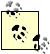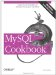# Adding a Temporal Interval to a Time

5.14.1 Problem

You want to add a given number of seconds to a time, or to add two time values.

5.14.2 Solution

Use TIME_TO_SEC( ) as necessary to make sure all values are represented in seconds, then add them. The result will be in seconds; use SEC_TO_TIME( ) if you want to convert back to a time value.

5.14.3 Discussion

The primary tools for performing time arithmetic are TIME_TO_SEC( ) and SEC_TO_TIME( ), which convert between TIME values and seconds. To add an interval value in seconds to a TIME value, convert the TIME to seconds so that both values are represented in the same units, add the values together, and convert the result back to a TIME. For example, two hours is 7200 seconds (2*60*60), so the following query adds two hours to each t1 value in the time_val table:

```mysql> SELECT t1,
-> SEC_TO_TIME(TIME_TO_SEC(t1) + 7200) AS 't1 plus 2 hours'
-> FROM time_val;
+----------+-----------------+
| t1 | t1 plus 2 hours |
+----------+-----------------+
| 15:00:00 | 17:00:00 |
| 05:01:30 | 07:01:30 |
| 12:30:20 | 14:30:20 |
+----------+-----------------+```

If the interval itself is expressed as a TIME, it too should be converted to seconds before adding the values together. The following example calculates the sum of the two TIME values in the time_val table:

```mysql> SELECT t1, t2,
-> SEC_TO_TIME(TIME_TO_SEC(t1) + TIME_TO_SEC(t2)) AS 't1 + t2'
-> FROM time_val;
+----------+----------+----------+
| t1 | t2 | t1 + t2 |
+----------+----------+----------+
| 15:00:00 | 15:00:00 | 30:00:00 |
| 05:01:30 | 02:30:20 | 07:31:50 |
| 12:30:20 | 17:30:45 | 30:01:05 |
+----------+----------+----------+```

It's important to recognize that MySQL TIME values really represent elapsed time, not time of day, so they don't reset to 0 after reaching 24 hours. You can see this in the first and third output rows from the previous query. To produce time-of-day values, enforce a 24-hour wraparound using a modulo operation before converting the seconds value back to a TIME value. The number of seconds in a day is 24*60*60, or 86400, so to convert any seconds value s to lie within a 24-hour range, use the MOD( ) function or the % modulo operator like this:

```MOD(s,86400)
s % 86400```

The two expressions are equivalent. Applying the first of them to the time calculations from the preceding example produces the following result:

```mysql> SELECT t1, t2,
-> SEC_TO_TIME(MOD(TIME_TO_SEC(t1) + TIME_TO_SEC(t2), 86400)) AS 't1 + t2'
-> FROM time_val;
+----------+----------+----------+
| t1 | t2 | t1 + t2 |
+----------+----------+----------+
| 15:00:00 | 15:00:00 | 06:00:00 |
| 05:01:30 | 02:30:20 | 07:31:50 |
| 12:30:20 | 17:30:45 | 06:01:05 |
+----------+----------+----------+```The allowable range of TIME values is -838:59:59 to 838:59:59 (that is -3020399 to 3020399 seconds). When you add times together, you can easily produce a result that lies outside this range. If you try to store such a value into a TIME column, MySQL clips it to the nearest endpoint of the range.MySQL Cookbook
ISBN: 059652708X
EAN: 2147483647
Year: 2005
Pages: 412
Authors: Paul DuBois